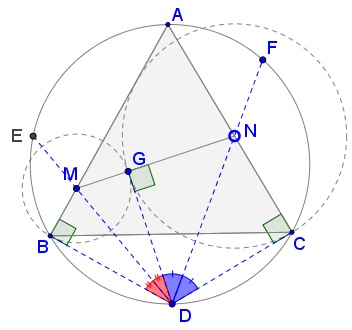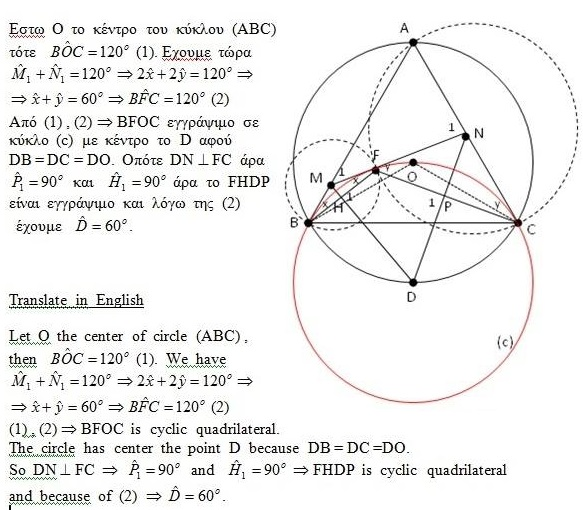# 60° Angle And Importance of Being The Other End of a Diameter

### Source### Problem### Solution 1

Observe that $AD\,$ is a diameter of $(ABC).\,$ It follows that $\angle ACD=\angle ABD=90^{\circ}.\,$ Also, $\angle CDB=120^{\circ}.\,$

$\mathbf{MN=BM+CN\,\Rightarrow\,\angle MDN=60^{\circ}}$

Let $G\in MN\,$ be such that $DG\perp MN.\,$ Consider circles $(M)=C(M,B)\,$ and $(N)=C(N,C).\,$To satisfy $MN=BM+CN,\,$ the two circles need to pass through $G\,$ where they touch each other, such that $BM=GM\,$ and $CN=GN.\,$ Note that the four triangles $BDM,\,$ $GDM,\,$ $GDN,\,$ and $CDN\,$ are right.

$BM=GM\,$ and $CN=GN\,$ is equivalent to
$\qquad\qquad\Delta BMD=\Delta GMD\,$ and $\Delta CND=\Delta GND$
which is equivalent to
$\qquad\qquad\angle BDM=\angle GDM\,$ and $\angle CDN=\angle GDN$
which implies
$\qquad\qquad\angle GDM+\angle GDN=60^{\circ}.$

$\mathbf{\angle MDN=60^{\circ}\,\Rightarrow\,MN=BM+CN}$

Consider circle $(D)\,$ through $B\,$ and $C.\,$ Let a tangent from $M\,$ to $(D)\,$ cross $AC\,$ at $N',\,$ with $G\,$ the tangency point.Then, $BM=GM\,$ and $CN'=GN',\,$ implying as above, that $\angle MDN'=60^{\circ}.\,$ It follows that $N'=N,\,$ $MN\,$ is tangent to $(D)\,$ at $G;\,$ $BM=GM\,$ and $CN=GN,\,$ and, therefore, $MN=BM+CN.$

### Solution 2### Acknowledgment

The above problem by Miguel Ochoa Sanchez was originally posted at the Peru Geometrico facebook group and appeared on my timeline. Solution 2 is by Stelios Karpathios and was been translated and posted by Takis Chronopoulos.

Thanos Kalogerakis has observed that the statement holds for isosceles triangles $ABC,\,$ with $AB=AC\,$ and $\displaystyle \angle MDN=\frac{1}{2}(180^{\circ}-\angle ABC).\,$ The proof appears to require only minor modifications.

• Angle Trisectors on Circumcircle
• Equilateral Triangles On Sides of a Parallelogram
• Pompeiu's Theorem
• Pairs of Areas in Equilateral Triangle
• The Eutrigon Theorem
• Equilateral Triangle in Equilateral Triangle
• Seven Problems in Equilateral Triangle
• Spiral Similarity Leads to Equilateral Triangle
• Parallelogram and Four Equilateral Triangles
• A Pedal Property in Equilateral Triangle
• Miguel Ochoa's van Schooten Like Theorem
• Two Conditions for a Triangle to Be Equilateral
• Incircle in Equilateral Triangle
• When Is Triangle Equilateral: Marian Dinca's Criterion
• Barycenter of Cevian Triangle
• Excircle in Equilateral Triangle
• Converse Construction in Pompeiu's Theorem
• Wonderful Trigonometry In Equilateral Triangle
• One More Property of Equilateral Triangles
• Van Khea's Quickie
• Equilateral Triangle from Three Centroids
•